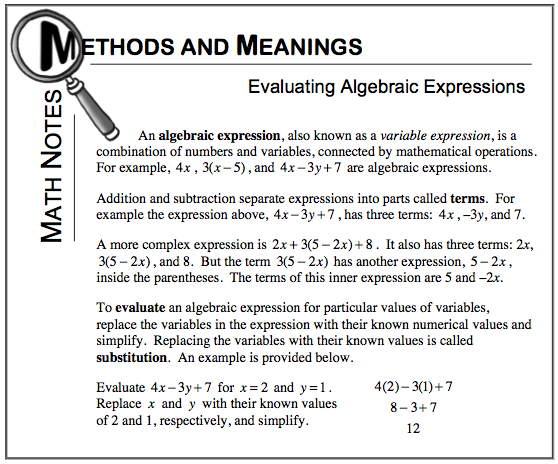### Home > CC1 > Chapter 6 > Lesson 6.2.1 > Problem6-75

6-75.

Evaluate the expressions below using $r = 3$ and $h = 5$. Homework Help ✎

1. $6 h - 4$

• Look at the Math Notes box below for help getting started on this problem.

• $6(5) − 4 = 26$

1. $8r+h$

• Remember that $8r$ means $8$ r’s or $8 × r$.

• $29$

1. $c \cdot r ^ { 2 }$

• Remember that the exponent (the two) means that you will multiply $r$ by itself.